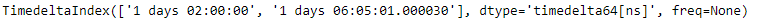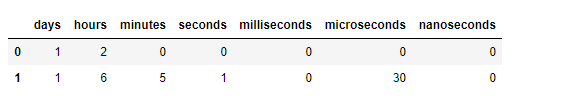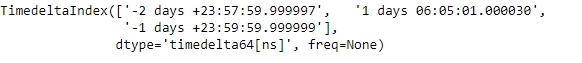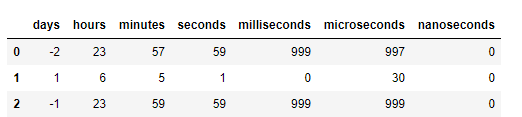# Python | Pandas TimedeltaIndex.components

• Last Updated : 29 Dec, 2018

Python is a great language for doing data analysis, primarily because of the fantastic ecosystem of data-centric python packages. Pandas is one of those packages and makes importing and analyzing data much easier.

Pandas` TimedeltaIndex.components` attribute return a dataframe of the components (days, hours, minutes, seconds, milliseconds, microseconds, nanoseconds) of the Timedeltas.

Syntax: TimedeltaIndex.components

Return : a DataFrame

Example #1: Use `TimedeltaIndex.components` attribute to return a dataframe of the components of the Timedeltas.

 `# importing pandas as pd``import` `pandas as pd`` ` `# Create the TimedeltaIndex object``tidx ``=` `pd.TimedeltaIndex(data ``=``[``'1 days 02:00:00'``, ``                                ``'1 days 06:05:01.000030'``])`` ` `# Print the TimedeltaIndex``print``(tidx)`

Output :Now we want to return a dataframe of the components of the tidx object.

 `# return a dataframe constructed from``# the components of the Timedelta``tidx.components`

Output :As we can see in the output, the `TimedeltaIndex.components` attribute has returned a dataframe constructed from the components of the Timedelta

Example #2: Use `TimedeltaIndex.components` attribute to return a dataframe of the components of the Timedeltas.

 `# importing pandas as pd``import` `pandas as pd`` ` `# Create the TimedeltaIndex object``tidx ``=` `pd.TimedeltaIndex(data ``=``[``'-1 days 2 min 3us'``, ``'1 days 06:05:01.000030'``,``                                                 ``'-1 days + 23:59:59.999999'``])`` ` `# Print the TimedeltaIndex``print``(tidx)`

Output :Now we want to return a dataframe of the components of the tidx object.

 `# return a dataframe constructed from``# the components of the Timedelta``tidx.components`

Output :As we can see in the output, the `TimedeltaIndex.components` attribute has returned a dataframe constructed from the components of the Timedelta

My Personal Notes arrow_drop_up